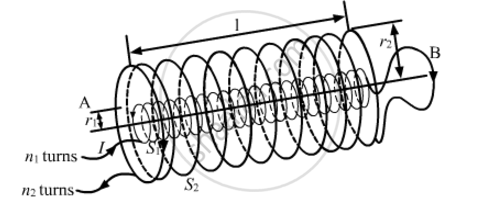# Two Long Coaxial Insulated Solenoids, S1 and S2 of Equal Lengths Are Wound One Over the Other as Shown in the Figure. a Steady Current "I" Flow Thought the Inner Solenoid S1 to the Other End B, Which is Connected to the Outer Solenoid S2 Through Which the Same Current "I" Flows in the Opposite Direction So as to Come Out at End A. - Physics

Two long coaxial insulated solenoids, S1 and S2 of equal lengths are wound one over the other as shown in the figure. A steady current "I" flow thought the inner solenoid S1 to the other end B, which is connected to the outer solenoid S2 through which the same current "I" flows in the opposite direction so as to come out at end A. If n1 and n2 are the number of turns per unit length, find the magnitude and direction of the net magnetic field at a point (i) inside on the axis and (ii) outside the combined system#### Solution

(i) The magnetic field due to a current carrying solenoid:

B=μ0ni

where, n = number of turns per unit length
i = current through the solenoid

Now, the magnetic field due to solenoid S1 will be in the upward direction and the magnetic field due to S2 will be in the downward direction (by right-hand screw rule).

Bnet=BS1BS2

Bnet=μ0n1Iμ0n2I

=μ0I(n1n2)

In the upward direction

(ii) The magnetic field is zero outside a solenoid.

Concept: Solenoid and the Toroid - the Solenoid
Is there an error in this question or solution?
2013-2014 (March) Delhi Set 1

Share